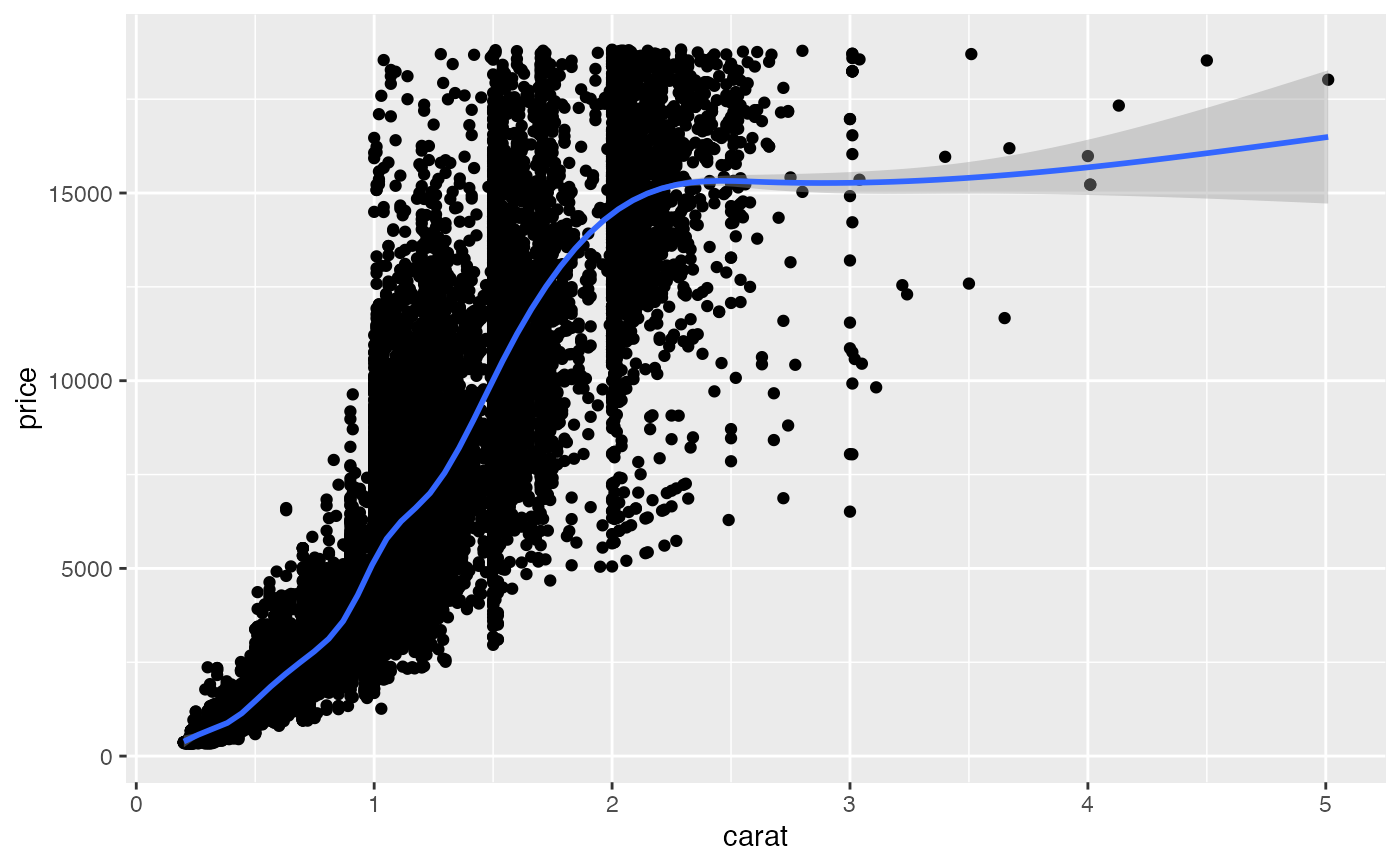For use in model formulas, natural cubic spline as in splines::ns but with knot positions chosen using k-means rather than quantiles. Automatically uses less knots if there are insufficient distinct values.

well_knotted_spline(x, n_knots, verbose = TRUE)

## Arguments

x The predictor variable. A numeric vector. Number of knots to use. If TRUE, produce a message about the knots chosen.

## Value

A matrix of predictors, similar to ns.

This function supports "safe prediction" (see makepredictcall). Original knot locations will be used for prediction with predict.

## Details

Wong (1982, 1984) showed the asymptotic density of k-means in 1 dimension is proportional to the cube root of the density of x. Compared to using quantiles (the default for ns), choosing knots using k-means produces a better spread of knot locations if the distribution of values is very uneven.

k-means is computed in an optimal, deterministic way using Ckmeans.1d.dp.

Wong, M. (1982). Asymptotic properties of univariate sample k-means clusters. Working paper #1341-82, Sloan School of Management, MIT. https://dspace.mit.edu/handle/1721.1/46876

Wong, M. (1984). Asymptotic properties of univariate sample k-means clusters. Journal of Classification, 1(1), 255–270. https://doi.org/10.1007/BF01890126

ns, makepredictcall

## Examples

lm(mpg ~ well_knotted_spline(wt,3), data=mtcars)
#> wt range 1.513 5.424 knots 2.199364 3.485556 5.339667#>
#> Call:
#> lm(formula = mpg ~ well_knotted_spline(wt, 3), data = mtcars)
#>
#> Coefficients:
#>                 (Intercept)  well_knotted_spline(wt, 3)1
#>                       32.13                       -17.46
#> well_knotted_spline(wt, 3)2  well_knotted_spline(wt, 3)3
#>                      -17.82                       -26.07
#> well_knotted_spline(wt, 3)4
#>                      -15.17
#>
# When insufficient unique values exist, less knots are used
lm(mpg ~ well_knotted_spline(gear,3), data=mtcars)
#> gear range 3 5 knots 3.6875#>
#> Call:
#> lm(formula = mpg ~ well_knotted_spline(gear, 3), data = mtcars)
#>
#> Coefficients:
#>                   (Intercept)  well_knotted_spline(gear, 3)1
#>                       16.1067                        14.8746
#> well_knotted_spline(gear, 3)2
#>                        0.9145
#>
library(ggplot2)
ggplot(diamonds, aes(carat, price)) +
geom_point() +
geom_smooth(method="lm", formula=y~well_knotted_spline(x,10))
#> x range 0.20 5.01 knots 0.3084627 0.4023654 0.5362350 0.7209752 0.8934446 1.0360370 1.2324172 1.5597639 2.0635750 2.6274262